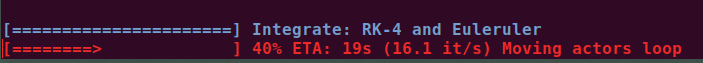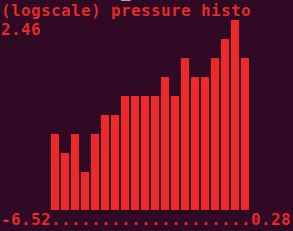# utils

Utilities submodule.

## ProgressBar

class vedo.utils.ProgressBar(start, stop, step=1, c=None, bold=True, italic=False, title='', ETA=True, width=25, char='━', char_back='─')[source]

Bases: `object`

Class to print a progress bar with optional text message.

Example
```import time
pb = ProgressBar(0,400, c='red')
for i in pb.range():
time.sleep(.1)
pb.print('some message')
```len()[source]

Return the number of steps.

print(txt='', counts=None, c=None)[source]

Print the progress bar and optional message.

range()[source]

Return the range iterator.

## cameraFromNeuroglancer

vedo.utils.cameraFromNeuroglancer(state, zoom=300)[source]

Define a `vtkCamera` from a neuroglancer state dictionary.

Parameters
• state (dict) – an neuroglancer state dictionary.

• zoom (float) – how much to multiply zoom by to get camera backoff distance default = 300 > ngl_zoom = 1 > 300 nm backoff distance.

Returns

a vtk camera setup that matches this state.

Return type

vtk.vtkCamera

## cameraFromQuaternion

vedo.utils.cameraFromQuaternion(pos, quaternion, distance=10000, ngl_correct=True)[source]

Define a `vtkCamera` with a particular orientation.

Parameters
• pos (np.array, list, tuple) – an iterator of length 3 containing the focus point of the camera

• quaternion (np.array, list, tuple) – a len(4) quaternion (x,y,z,w) describing the rotation of the camera such as returned by neuroglancer x,y,z,w all in [0,1] range

• distance (float) – the desired distance from pos to the camera (default = 10000 nm)

Returns

a vtk camera setup according to these rules.

Return type

vtk.vtkCamera

## cart2pol

vedo.utils.cart2pol(x, y)[source]

2D Cartesian to Polar coordinates conversion.

## cart2spher

vedo.utils.cart2spher(x, y, z)[source]

3D Cartesian to Spherical coordinate conversion.

## dotdict

class vedo.utils.dotdict(*args, **kwargs)[source]

Bases: `dict`

A dictionary supporting dot notation.

Example

```dd = dotdict({"a": 1,
"b": {"c": "hello",
"d": [1, 2, {"e": 123}]}
}
)
dd.update({'k':3})
dd.g = 7
print("k=", dd.k)           # k= 3
print(dd.b.c)               # hello
print(isinstance(dd, dict)) # True
print(dd.lookup("b.d"))     # [1, 2, {"e": 123}]
```
lookup(dotkey)[source]

Lookup value in a nested structure with a single key, e.g. “a.b.c”.

## geometry

vedo.utils.geometry(obj, extent=None)[source]

Apply the `vtkGeometryFilter`. This is a general-purpose filter to extract geometry (and associated data) from any type of dataset. This filter also may be used to convert any type of data to polygonal type. The conversion process may be less than satisfactory for some 3D datasets. For example, this filter will extract the outer surface of a volume or structured grid dataset.

Returns a `Mesh` object.

Parameters

extent (list) – set a [xmin,xmax, ymin,ymax, zmin,zmax] bounding box to clip data.

## grep

vedo.utils.grep(filename, tag, firstOccurrence=False)[source]

Greps the line that starts with a specific tag string inside the file.

## humansort

vedo.utils.humansort(l)[source]

Sort in place a given list the way humans expect.

NB: input list is modified

E.g. [‘file11’, ‘file1’] -> [‘file1’, ‘file11’]

## isSequence

vedo.utils.isSequence(arg)[source]

Check if input is iterable.

## linInterpolate

vedo.utils.linInterpolate(x, rangeX, rangeY)[source]

Interpolate linearly the variable x in rangeX onto the new rangeY. If x is a 3D vector the linear weight is the distance to the two 3D rangeX vectors.

E.g. if x runs in rangeX=[x0,x1] and I want it to run in rangeY=[y0,y1] then y = linInterpolate(x, rangeX, rangeY) will interpolate x onto rangeY.

## mag

vedo.utils.mag(v)[source]

Get the magnitude of a vector or array of vectors.

## mag2

vedo.utils.mag2(v)[source]

Get the squared magnitude of a vector or array of vectors.

## makeBands

vedo.utils.makeBands(inputlist, numberOfBands)[source]

Group values of a list into bands of equal value.

Parameters

numberOfBands (int) – number of bands, a positive integer > 2.

Returns

a binned list of the same length as the input.

## numpy2vtk

vedo.utils.numpy2vtk(arr, dtype=None, deep=True, name='')[source]

Convert a numpy array into a vtkDataArray. Use dtype=’id’ for vtkIdTypeArray objects.

## orientedCamera

vedo.utils.orientedCamera(center=(0, 0, 0), upVector=(0, 1, 0), backoffVector=(0, 0, 1), backoff=1)[source]

Generate a `vtkCamera` pointed at a specific location, oriented with a given up direction, set to a backoff.

## pointIsInTriangle

vedo.utils.pointIsInTriangle(p, p1, p2, p3)[source]

Return True if a point is inside (or above/below) a triangle defined by 3 points in space.

## pointToLineDistance

vedo.utils.pointToLineDistance(p, p1, p2)[source]

Compute the distance of a point to a line (not the segment) defined by p1 and p2.

## pol2cart

vedo.utils.pol2cart(rho, theta)[source]

2D Polar to Cartesian coordinates conversion.

## precision

vedo.utils.precision(x, p, vrange=None, delimiter='e')[source]

Returns a string representation of x formatted with precision p.

Parameters

vrange (float) – range in which x exists (to snap x to ‘0’ if below precision).

## printHistogram

vedo.utils.printHistogram(data, bins=10, height=10, logscale=False, minbin=0, horizontal=False, char='▉', c=None, bold=True, title='Histogram')[source]

Ascii histogram printing. Input can also be `Volume` or `Mesh`. Returns the raw data before binning (useful when passing vtk objects).

Parameters
• bins (int) – number of histogram bins

• height (int) – height of the histogram in character units

• logscale (bool) – use logscale for frequencies

• minbin (int) – ignore bins before minbin

• horizontal (bool) – show histogram horizontally

• char (bool) – character to be used

• c (str,int) – ascii color

• char – use boldface

• title (str) – histogram title

Example
```from vedo import printHistogram
import np as np
d = np.random.normal(size=1000)
data = printHistogram(d, c='blue', logscale=True, title='my scalars')
data = printHistogram(d, c=1, horizontal=1)
print(np.mean(data)) # data here is same as d
```## printInfo

vedo.utils.printInfo(obj)[source]

Print information about a vtk object.

## resampleArrays

vedo.utils.resampleArrays(source, target, tol=None)[source]

Resample point and cell data of a dataset on points from another dataset. It takes two inputs - source and target, and samples the point and cell values of target onto the point locations of source. The output has the same structure as the source but its point data have the resampled values from target.

Parameters

tol (float) – set the tolerance used to compute whether a point in the target is in a cell of the source. Points without resampled values, and their cells, are be marked as blank.

## roundToDigit

vedo.utils.roundToDigit(x, p)[source]

Round a real number to the specified number of significant digits.

## spher2cart

vedo.utils.spher2cart(rho, theta, phi)[source]

3D Spherical to Cartesian coordinate conversion.

## trimesh2vedo

vedo.utils.trimesh2vedo(inputobj, alphaPerCell=False)[source]

Convert `Trimesh` object to `Mesh(vtkActor)` or `Assembly` object.

## vector

vedo.utils.vector(x, y=None, z=0.0, dtype=<class 'numpy.float64'>)[source]

Return a 3D numpy array representing a vector.

If y is `None`, assume input is already in the form [x,y,z].

## vedo2trimesh

vedo.utils.vedo2trimesh(mesh)[source]

Convert `vedo.Mesh` to `Trimesh.Mesh` object.

## versor

vedo.utils.versor(x, y=None, z=0.0, dtype=<class 'numpy.float64'>)[source]

Return the unit vector. Input can be a list of vectors.

## vtk2numpy

vedo.utils.vtk2numpy(varr)[source]

Convert a vtkDataArray or vtkIdList into a numpy array

## vtkCameraToK3D

vedo.utils.vtkCameraToK3D(vtkcam)[source]

Convert a `vtkCamera` object into a 9-element list to be used by K3D backend.

Output format is: [posx,posy,posz, targetx,targety,targetz, upx,upy,upz]